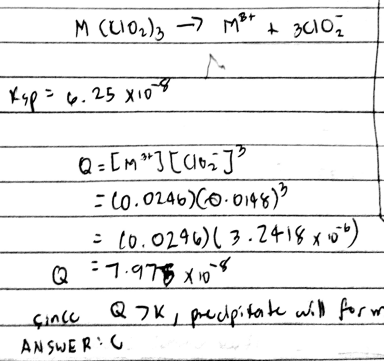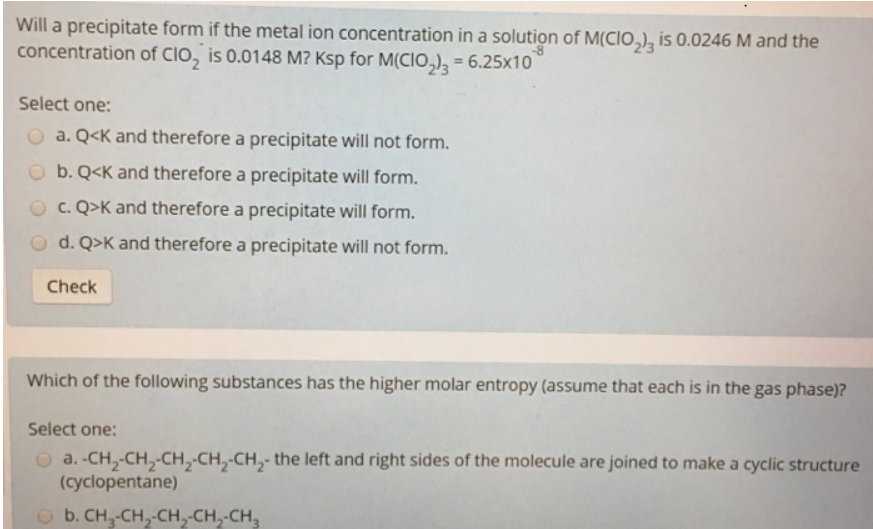# Problem: Will a precipitate form if the metal ion concentration in a solution of M(CIO2)3 is 0.0246 M and the concentration of CIO2 is 0.0148 M? Ksp for M(ClO2)3 = 6.25x10-8 Which of the following substances has the higher molar entropy (assume that each is in the gas phase)?

###### FREE Expert Solution88% (295 ratings)###### Problem Details

Will a precipitate form if the metal ion concentration in a solution of M(CIO2)3 is 0.0246 M and the concentration of CIO2 is 0.0148 M? Ksp for M(ClO2)3 = 6.25x10-8

Which of the following substances has the higher molar entropy (assume that each is in the gas phase)?